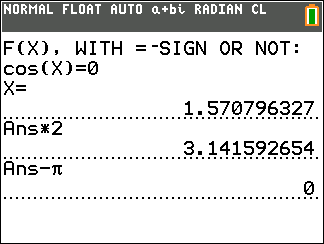Basics Archives Community Services ProgrammingHome :: Archives :: File Archives :: EquationEquation

FILE INFORMATION

equation.zip
 Filename equation.zip (Download) Title Equation Description EQUATION: A very simple program that uses the Solve(-function to give one answer to your equations in X. Enter the equation with, or without, a =-sign. For example, 2x-45=0, or sin(x)=1/sqrt2. Or 2x-45, or sin(x)-1/sqrt2. The program can handle even the tricky equations, without you having to make a guess for the root. EQUATN4: This program is an expansion of EQUATION, that can give four answers to for example a fourth degree equation like, 117x^4-20x^3-390x^2+20x+273=0. Or a statement like, abs((x+2)(x-5))=3. If there are less than four answers to the expression, the programs stops with an, ERROR: NO SIGN CHANGE. Just press 1: QUIT then. The answers are in memories A, B, C, and D. Author Anders Tiberg (anders.tiberg@telia.com) Category TI-84 Plus C Silver Edition/CE BASIC Math Programs File Size 7,254 bytes File Date and Time Sat Aug 11 18:36:48 2018 Documentation Included? Yes

SCREEN SHOTSREVIEWS

There are no reviews for this file.

LEAVE FEEDBACK

Questions, comments, and problems regarding the file itself should be sent directly to the author(s) listed above.

Report inappropriate or miscategorized file (requires an account; or you may email us directly)

 Bad Good 1  2  3  4  5  6  7  8  9  10

ARCHIVE CONTENTS

Archive Contents
Name Size
EQUATION.8xp   307
EQUATION.rtf   1335
EQUATN4-1.png   3148
EQUATN4-2.png   2573
EQUATN4.8xp   482